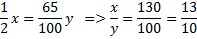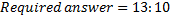## Percentage For SBI PO : Set -17

1) The sum of 45% of a number and 20% of the same number is 4056. What is 85% of that number?

a) 5043

b) 5430

c) 5034

d) 5340

e) None of these

e)

45% of (x) + 25% of (x) = 4056

X = 6240

85% of (6240) = 5304

2) The sum of 15% of a positive number and 10% of the same number is 70. What is twice of that number?

a) 440

b) 280

c) 560

d) 140

e) None of these

c)

Sum of numbers = 15% of (x) + 10% of (x) = 70

X = 280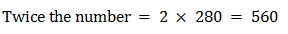3) If a numerator of a fraction is increased by 400% and the denominator is increased by 500%, the resultant fraction is 10/21. What was the original fraction?

a) 5/12

b) 8/13

c) 17/14

d) 4/7

e) None of these

d)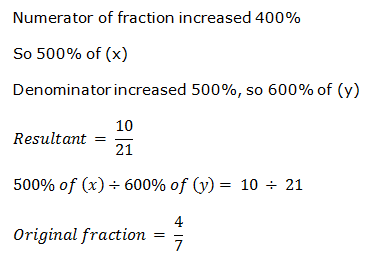4) 10% of 20% of  of a number is 102. What is the number?

a) 10200

b) 10460

c) 10540

d) 10720

e) 10860

a)

10% of 20% of ½ of a number is 102.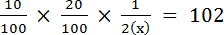5) The difference between 58% of a number and 39% of the same number is 247. What is 62% of that number?

a) 1300

b) 806

c) 754

d) 1170

e) None of these

b)

58% of x-39% of x=247

-> 0.58x-0.39x=247->x=1300

Required Answer = 0.67 * 1300 =806

6) There are 1240 employees in an organization. Out of which 25% got promoted. How many such employees are there who got promotion?

a) 398

b) 345

c) 310

d) 372

e) None of these

C)

According to question,

1240*25/100=310

7) Bina’s monthly income is 90% of Anita’s monthly income. The total of both their monthly incomes is Mr. Sen’s monthly income. Mr. Sen’s annual income is Rs. 775200. What is Bina’s monthly income? (In some cases annual income is asked and in some case monthly income is asked)

a) Rs. 34000

b) Rs. 36000

c) Rs. 30600

d) Rs. 30000

e) None of these

C)

Bina’s monthly income (B) = 90/100 Anitha’s monthly income (A)

A+B = Sen’s monthly income(S)

Sen’s monthly income =775200/12=Rs.64600

According to question

A+0.9A=64600->1.9A=64600-> A = Rs.34000

Therefore, Bina’s Monthly income (B) = 64600-34000= Rs.30600

8) The product of 5% of a positive number and 3% of the same number is 504.6. What is half of that number?

a) 290

b) 340

c) 680

d) 580

e) None of these

a)

According to question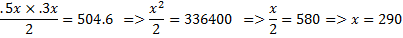9) Three-fourth of a number is equal to 60% of another number. What is the difference between the numbers?

a) 18

b) 32

c) 24

d) Cannot be determined

e) None of these

d)

According to question,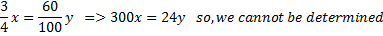10) Half of a number is equal to 65% of another number. What is the ratio between the first number and the second number respectively?

a) 10:13

b) 8:13

c) 13:8

d) 13:10

e) None of these